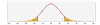# Confidence Interval Calculator For Two Proportions

Confidence interval calculator for the difference between two proportions with calculation steps, using the normal distribution approximation.#### Two proportions confidence interval calculator

When using the sample data, we know the different between the sample proportions but we don't know the true value of the different between the population's proportions. Instead, we may treat the population's proportions as random variables and calculate the confidence interval.
First, we need to define the confidence level which is the required certainty level that the true value will be in the confidence interval Researchers commonly use a confidence level of 0.95.
We use the normal approximation.

##### Two proportions confidence interval formula
 CI = p̂1 - p̂2 ± Z1-α/2 √( p̂1(1 - p̂1) + p̂2(1 - p̂2) ) n1 n2
##### Two proportions margin of error formula

MOE = Z1-α/2*√(σ21 + σ22)

 MOE = Z1-α/2√( p̂1(1 - p̂1) + p̂2(1 - p̂2) ) n1 n2
##### How to use the two proportion confidence interval calculator?
1. Confidence level - The certainty level that the true value of the estimated parameter will be in the confidence interval, usually 0.95.
2. Rounding - how to round the results?
When a resulting value is larger than one, the tool rounds it, but when a resulting value is less than one the tool displays the significant figures.
3. Sample sizes (n1, n2) - the number of subjects.
4. Sample proportion (p̂1, p̂2) or #successes (x1, x2): If the value you entered is between 0 and 1 - the calculator assumed that you enter proportion (proabability).
When the value is 1, or larger - the calculator assumed that you enter the number of successes.
For example, when the sample size is 12 if you enter 3 the tool assumes 3 successes, x=3, and will calculate p̂ = 3/12 = 0.25.
If you enter 0.25, the tool assumes p̂ = 3/12 = 0.25, and the confidence interval will be the same.
5. Continuity correction - since we use the continuous normal distribution to approximate the discrete binomial distribution, the Continuity correction support results more similar to the binomial distribution.
If you are a student, don't use the continuity correction.